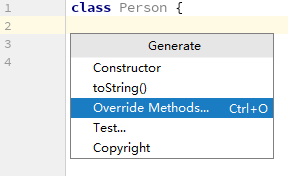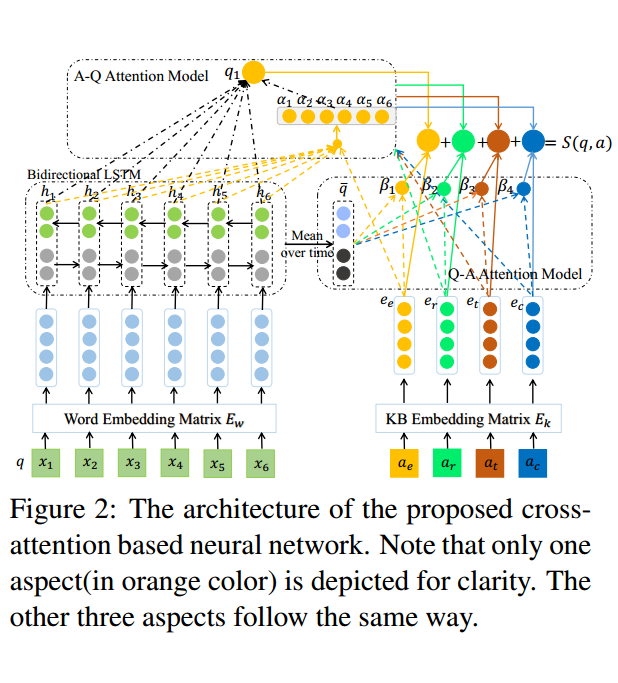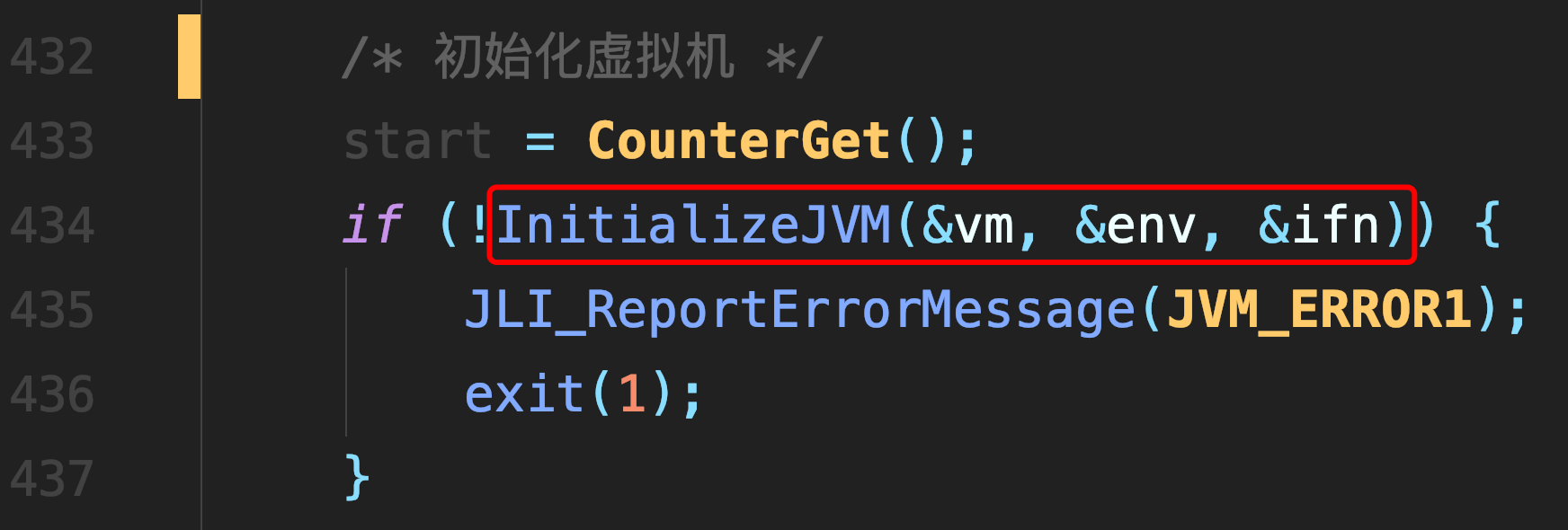# Populating Next Right Pointers in Each Node I, II

### Populating Next Right Pointers in Each Node I, II

#### 题目I

Given a binary tree

``````    struct TreeLinkNode {
}
``````

Populate each next pointer to point to its next right node. If there is no next right node, the next pointer should be set to `NULL`.

Initially, all next pointers are set to `NULL`.

Note:

• You may only use constant extra space.
• You may assume that it is a perfect binary tree (ie, all leaves are at the same level, and every parent has two children).

For example,
Given the following perfect binary tree,

``````         1
/  \       2    3
/ \  / \     4  5  6  7
``````

After calling your function, the tree should look like:

``````         1 -> NULL
/  \
2 -> 3 -> NULL
/ \  / \
4->5->6->7 -> NULL``````

#### 思路

``````/**
* Definition for binary tree with next pointer.
*  int val;
*  TreeLinkNode(int x) : val(x), left(NULL), right(NULL), next(NULL) {}
* };
*/
class Solution {
public:
if(root == NULL) return ;

while(temp != NULL) {
curLne = temp;
while(curLne != NULL) {
if(curLne->left != NULL)
curLne->left->next = curLne->right;

if(curLne->next != NULL && curLne->right != NULL)
curLne->right->next = curLne->next->left;

curLne = curLne->next;
}

temp = temp->left;
}
}
};``````

### 题目II

Follow up for problem “Populating Next Right Pointers in Each Node“.

What if the given tree could be any binary tree? Would your previous solution still work?

Note:

• You may only use constant extra space.

For example,
Given the following binary tree,

``````        1
/  \      2    3
/ \    \    4   5    7
``````

After calling your function, the tree should look like:

``````        1 -> NULL
/  \
2 -> 3 -> NULL
/ \    \
4-> 5 -> 7 -> NULL
``````

### 思路

``````class Solution {
public:
if(root == NULL) return;
while(root) {
start = findStart(root);
current = start;

while(current) {
current->next = findNext(root, current);
current = current->next;
}

root = start;
}
}

private:
while(root) {
if(root->left == current) {
if(root->right != NULL) return root->right;
root = root->next;
while(root) {
if(root->left != NULL) return root->left;
if(root->right != NULL) return root->right;
root = root->next;
}
return NULL;
}
if(root->right == current) {
root = root->next;
while(root) {
if(root->left != NULL) return root->left;
if(root->right != NULL) return root->right;
root = root->next;
}
return NULL;
}
root = root->next;
}
return NULL;
}

if(root == NULL) return NULL;
while(root) {
if(root->left != NULL) return root->left;
if(root->right != NULL) return root->right;
root = root->next;
}
return NULL;
}
};``````

##### 热门推荐
•浏览(655)
•浏览(565)
•浏览(548)
•浏览(488)
•浏览(434)
•浏览(424)
•浏览(381)
•浏览(367)
•浏览(362)
•浏览(358)